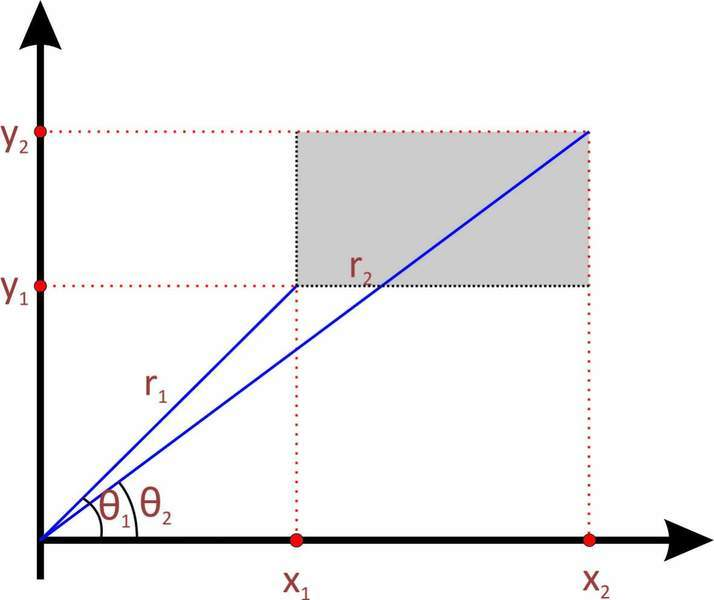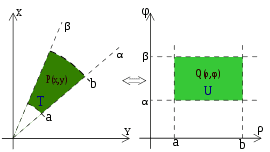# Cartesian and Polar coordinate system increments

we know that for any (x, y) in Cartesian system, there is such (r, θ) in Polar system, so

x = r * cosθ and
y = r * sinθ

how you can calculate what corresponds to (Δx, Δy) in polar system?

how come Δx * Δy = r * Δr * Δθ?

Maybe this is very stupid question and has obvious answer, but I haven't been practicing higher math since the university (8 years since graduation), but now I need this proof to prove that for normal distribution the sum of all probabilities in (-∞; ∞) equals to 1.

I guess this is which area on polar coordinate system corresponds to the area of Δx * Δy...
But which and how you calculate that?

I attach graphical presentation of the problem. The shadowed area needs to be presented in polar coordinates...#### Attachments

I like Serena
Homework Helper
Welcome to PF, XYZ313!Your problem statement uses the word "correspond".
Actually, in polar coordinates you would not use the rectangle you have in your drawing, since it won't help you.
What you would do is use something that is approximately (in the first order) a rectangle that aligns with the polar coordinates.
This is shown in the leftmost picture of this drawing (just one I plucked somewhere to illustrate my point):Can you tell what the lengths of sides of the figure are?

Furthermore, your equation Δx * Δy = r * Δr * Δθ is not correct.
These values are not equal.

lurflurf
Homework Helper
That is from calculus it is called Jacobian determinant.
It works linearizing the change of variable (x,y)->(u,v)
dudv=(uxdx+uydy)(vxdx+vydy)
=(uxvy-uyvx)dxdy

Simon Bridge
Homework Helper
Looks like:
$$dx.dy → r.dr.d\theta$$

it's approximate except where the area is very small, like you use in integration ...

dx.dy is a very small area, so small it fits in all the weird corners of whatever your shape is. The idea of integration is that you divide your shape into lots of these and then count how many you have and the total is the area of the shape.

for some shapes it is easier on the math to turn them into lots of little wedge-segments like the one in the Serenaphile's post. If the area is very small then it is very nearly a wee rectangle with a base of r.dθ and a height dr which gives a total area of r.dr.dθ

the Jacobean, as mentioned, works for any change in coordinates but is pretty abstract. The wedge helps you understand better.

For your rectangle, it should be clear that for large ΔxΔy the polar representation will depend on where it is.

HallsofIvy
Homework Helper
Another, more advanced, way to look at it. If you have a surface defined by parametric equations, x= f(u,v), y= g(u,v), z= h(u,v), then you can write the "position vector" for any point on the surface as $\vec{r}= f(u,v)\vec{i}+ g(u,v)\vec{j}+ h(u,v)\vec{k}$. The derivatives with respect to the two parameters,
$$\vec{r}_u= f_u\vec{i}+ g_u\vec{j}+ h_u\vec{k}$$
and
$$\vec{r}_v= f_v\vec{i}+ g_v\vec{j}+ h_v\vec{k}$$
lie in the tangent plane to the surface and describe how "measurement" changes in each parameter direction. Their cross product, $\vec{r}_u\times\vec{r}_v$, is perpendicular to the surface at each point and its length gives the differential of surface area of the surface: $|\vec{r}_u\times\vec{r}_v|dudv$.

In particular, we can write the xy-plane as $\vec{r}(x,y)= x\vec{i}+ y\vec{j}$ or, in polar coordinates, $\vec{r}(r, \theta)= r cos(\theta)\vec{i}+ r sin(\theta)\vec{j}$. The derivatives with respect to the parameters are:
$$\vec{r}_r= cos(\theta)\vec{i}+ sin(\theta)\vec{j}$$
and\
$$\vec{r}_\theta= -r sin(\theta)\vec{i}+ r cos(\theta)\vec{j}$$

The cross product of those two vectors is
$$\left|\begin{array}{ccc}\vec{i} & \vec{j} & \vec{k} \\ cos(\theta) & sin(\theta) & 0 \\ -r sin(\theta) & r cos(\theta) & 0 \end{array}\right|= 0\vec{i}+ 0\vec{j}+ (rcos^2(\theta)+ rsin^2(\theta))\vec{k}= r\vec{k}$$
So that the differential of area is $r drd\theta$.

Thank you all!

I like Serena: yes you are right, I should have used the word "correspond";

Simon Bridge: yes, the changes are very small, just wrote with delta to make it more readable.

Simon Bridge: SerenaphileI like Serena: which Serena is that? Gossip Girl or Gomez :)?

Thank you again I like Serena, lurflurf, Simon Bridge, HallsofIvy!

I like Serena
Homework Helper
Simon Bridge: SerenaphileI like Serena: which Serena is that? Gossip Girl or Gomez :)?

Thank you again I like Serena, lurflurf, Simon Bridge, HallsofIvy!

First time I've been called Serenaphile. I'll remember and use that!Neither Serena, nor Serena "Black Booty" Williams.

It's this one:
http://masterofmagic.info/node/19 [Broken]

[PLAIN]http://masterofmagic.info/sites/default/files/serena.gif [Broken]

It's from a very old computer game.--I like Serenaphile

Last edited by a moderator: